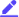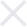Location: Home > Games > Education > Chemical Formula Quiz# Chemical Formula Quiz Apk v1.0.1

App Name: Chemical Formula Quiz
1.0.1 for Android Updated on Tue Oct 25 12:35:33 CST 20226.0The description of Chemical Formula Quiz
Chemical & Chemistry Formula Quiz Game

This collection of more than 3000 chemical formula quiz deals with the basic concepts of organic chemistry formula and other chemical formula trivia. Topics include simplest and molecular formulas, mass percent composition and naming compounds.

The molecular formula or chemistry formulas are an expression of the number and type of atoms that are present in a single molecule of a substance. It represents the actual formula of a molecule. Subscripts after element symbols represent the number of atoms. If there is no subscript, it means one atom is present in the compound.

The empirical formula is also known as the simplest formula. The chemical formula is the ratio of elements present in the compound. The subscripts in the formula are the numbers of atoms, leading to a whole number ratio between them.

Chemistry trivia quiz game exemple
The molecular formula of glucose is C6H12O6. One molecule of glucose contains 6 atoms of carbon, 12 atoms of hydrogen and 6 atoms of oxygen.

These are ten multiple choice questions covering chemistry in this chemistry game. Topics include simplest and molecular formulas and naming compounds.

Also study the structure, properties, composition, reactions, and preparation of carbon-containing compounds in this chemistry game.
Now it's up to you :)

Category: Education Publisher: Jamal ouali Requirements: Andriod 5.0+ Publish Date: Tue Oct 25 12:35:33 CST 2022

### Tags

Chemical Formula Quiz 1.0.1 Update
`Updated on Tue Oct 25 12:35:33 CST 2022Bug Fixes`

### Reviews (0)Write ReviewNo content~Mods Name …
Creation time:33.7MB
Version :
1.0.1
Version Code :
228006
Language :
EN AM AR AS BE BG BN BS CA CS EN AM AR ASs BE BG BN BS CA CS EN AM AR AS BE BG BN BS CA CS
Permission :
EN AM AR AS BE BG BN BS CA CS EN AM AR ASs BE BG BN BS CA CS EN AM AR AS BE BG BN BS CA CS